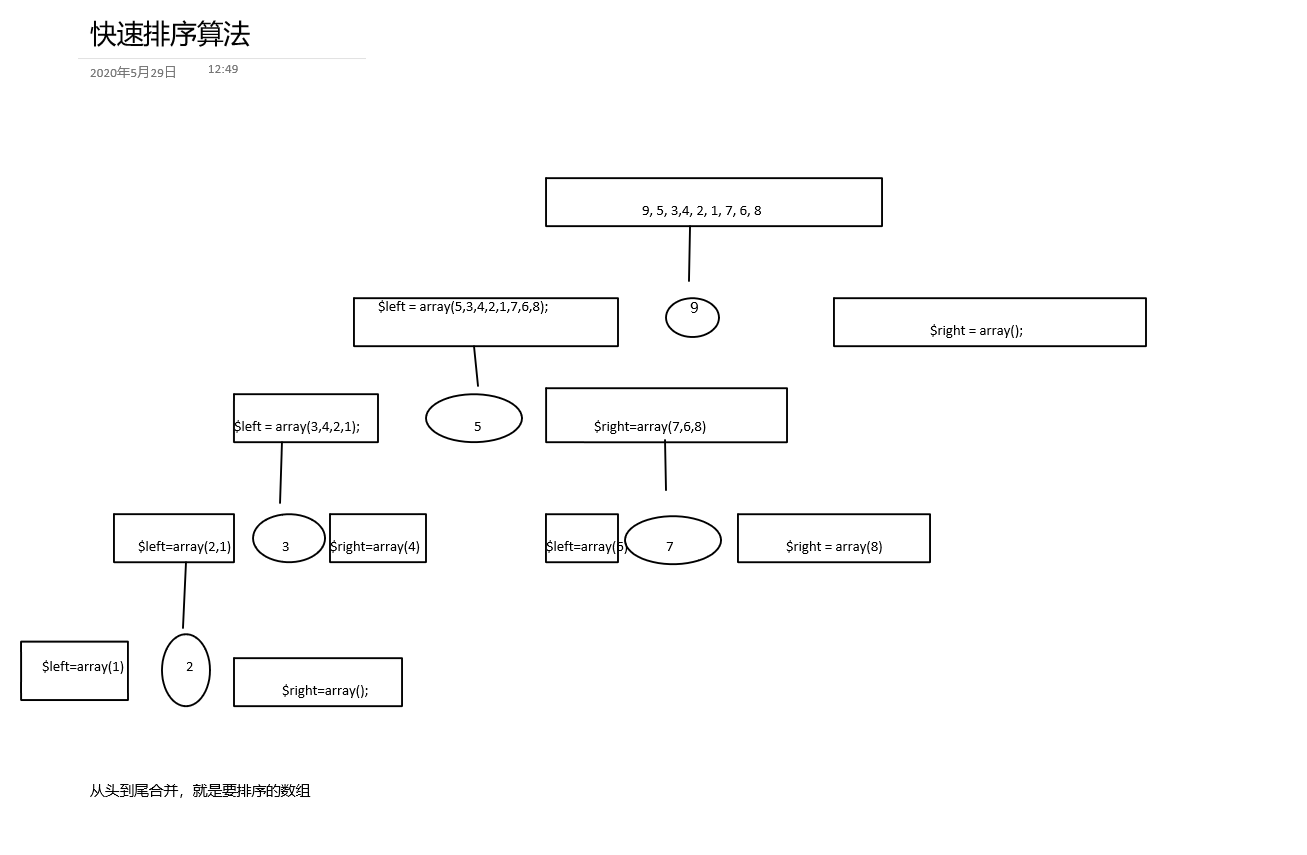# 排序算法-快速排序

## 快速排序``````<?php

\$arr = array(9, 5, 3,4, 2, 1, 7, 6, 8);

function quick_sort(\$arr)
{
if (!is_array(\$arr)) {
return false;
}

\$length = count(\$arr);
//为空和只有一个元素，都直接返回
if (\$length <= 1) {
return \$arr;
}

\$left = \$right = array();
\$key = \$arr;
for (\$i = 1; \$i < \$length; \$i++) {
if (\$arr[\$i] < \$key) {
\$left[] = \$arr[\$i];
} else {
\$right[] = \$arr[\$i];
}
}

\$left = quick_sort(\$left);
\$right = quick_sort(\$right);
return array_merge(\$left, array(\$key), \$right);
}

print_r(quick_sort(\$arr));``````